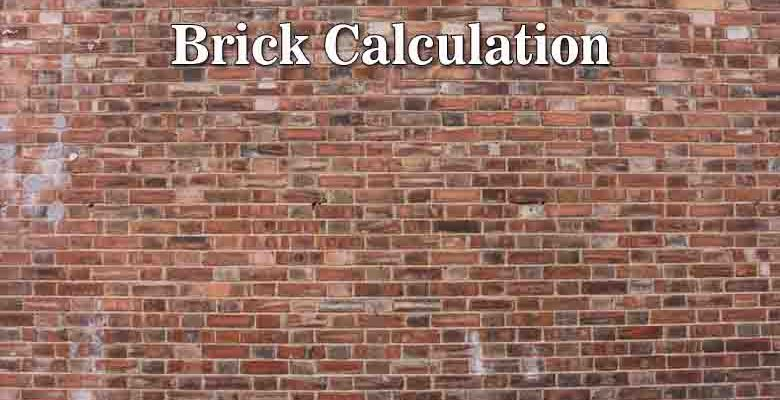# Brick Wall area with Brick perfect Quantity Calculation 23

## Brick area and cost calculation

Brick wall area easy calculation with brick cost.

## How to use this table for getting Brick, wall area, and cost

• First add value of Room parameter meter, feet or inch in the input box,
• Then add value of Room door area in meter, feet or inch in the input box,
• Then add value of Room window area in meter, feet or inch in the input box,
• Then add value of Room wall height in meter, feet or inch in the input box,
• Then add value of Room wall width in meter, feet or inch in the input box,
• Then Click on Wall area button for getting area of Room in square meter, square feet or square inch,
• Then add value of Brick Length in meter, feet or inch in the input box,
• Then add value of Brick Height in meter, feet or inch in the input box,
• Then add value of horizontal plaster between brick add Height in meter, feet or inch in the input box,
• Then add value of vertical plaster between brick add Length in meter, feet or inch in the input box,
• Then Click on 1 Brick or Block area button for getting area of Room in square meter, square feet or square inch,
• Then Click on 1 Brick or Block Qty. button for getting total Brick quantity,
• Then add cost of one Brick rate in Rupees,
• Then Click on button of Brick Cost for getting total cost of room wall.
Single Wall area – volume – Brick Quantity perfect cost Details 22

## Brick Wall Volume with Brick or Block Quantity Calculation

Room parameter
Meter
Feet
Inch
Deduct Door Area:
Square Meter
Square Feet
Square Inch
Deduct Window Area:
Square Meter
Square Feet
Square Inch
Wall Height:
Meter
Feet
Inch
Wall Width:
Meter
Feet
Inch
Sq. Meter
Sq. Feet
Sq. Inch
Brick Length:
Meter
Feet
Inch
Brick Height:
Meter
Feet
Inch
horizontal plaster between brick add Height:
Meter
Feet
Inch
vertical plaster between brick add Length:
Meter
Feet
Inch
Square Meter
Square Feet
Square Inch
Brick Rate
Rate of 1 brick or Block in Rupees